Transcripted Summary

I want to show you some other useful things you can do with arrays such as having a dynamic array length when you aren't sure how big the array should be. And also using an accumulator to sum and average all elements in an array.We have a class here and we’re going to allow the user to enter the number of grades.

``````package chapter7;

/*
* Create a program that allows a user to enter any
* number of grades and provides them with their
* average score, as well as the highest and lowest scores.
*/

public static void main(String[] args){

}
}
``````

We don't know how many grades they're going to enter, so it's going to be very difficult to define this array.

But let's go ahead and just declare the array for starters.

``````public class Grades {

public static void main(String[] args){

}
}
``````

And then, inside of the `main` method we are going to ask the user how many grades they'd like to enter, and we'll use that number as the length of the array.

``````private static int grades[];

public static void main(String[] args){

System.out.println("How many grades would you like to enter?");
}
``````

Now I can go initialize grades with the length that the user enters.

``````private static int grades[];

public static void main(String[] args){

System.out.println("How many grades would you like to enter?");

}
``````

So now we have the value.

We didn't have to specify the array’s length when we first declared the array, but we've updated it now that we know how many grades we need to deal with.

Okay, so our program now wants to allow them to enter the grades.

So, let's make a call to a new method: `getGrades()`

``````public static void getGrades(){

}
``````

This method will ask the user to enter their grades. And to do that, of course we want to do it however many times that they ask us to.

So, let's say that there were 20 grades that needed to be done. Of course, we're going to want to use a loop for this.

We're not going to use the enhanced `for` loop this time because that type of loop works best when you're working with values that are already there. In this case, we have an empty array, we have no values, and we want to increment the counter.

So, we'll go ahead and use the traditional `for` loop.

``````public static void getGrades(){

}
}
``````

Notice now, I'm using `.length` and this is a property of arrays. You can use a `.length` to get how big that array is. I don't have to worry about what was passed into here. Since I have `grades` as this class variable, I can use it here.

So inside of here we'll go ahead and prompt the user and then store it in the array.

``````for(int i=0; i<grades.length; i++){

}
``````

So now we have the grades. The next thing we want to do is to sum up all of the grades.

Let's create a method that will sum all the grades up.

``````public static int calculateSum(){

}
``````

This time we do have values inside of our array, so it makes sense to use the enhanced `for` loop this time.

So, every element will be an `int`. We'll use the colon and we're going to iterate over the `grades` array.

``````public static int calculateSum(){
int sum = 0;

}
return sum;
}
``````

We are going to create this variable — `sum` — outside of the loop. Again, the reason why we're doing this is because of scope.

If I were to declare this `sum` inside of this `for` loop, then I wouldn't be able to return the `sum` outside of the `for` loop.

Inside of this `for` loop, we're going to accumulate the sum.

``````for(int grade : grades){
}
``````

And then we need to find the average score.

It asks us to do the average, the highest and the lowest, so we're going to make a new method for the average.

``````public static double calculateAverage(){

}
``````

To get that average is simply going to be the sum divided by the number of grades. So again, we'll use that `.length`.

``````public static double calculateAverage(){
}
``````

So here in our `main, we'll go ahead and print that out.

``````public static void main(String[] args){

System.out.println("How many grades would you like to enter?");

System.out.println("Average: " + String.format("%.2f", calculateAverage()));
}
``````

I use `String.format` to be able to format my numbers. So, this `calculateAverage` is going to give us a `double`. It could be a very long `double` with a lot of numbers after the decimal point.

You can use `String.format` not just for numbers. I also use it for Strings. I use it all the time, actually, in my real programming. So, I'll start using it from now on in this course. But this little percent sign (%) is used as a placeholder.

So, with this I'm saying, “As a placeholder (%), I'm going to give you some value — whatever I get from this (`calculateAverage()`). I want you to replace this placeholder with that value.” But since it's a number, I'm going to use `.2f``, meaning that it is a float number. And I only want you to give me two decimal places. I know, it's a little bit much. We'll see it more often. You'll get used to it.

Okay, so we have the average.

What else did they want us to do? The highest and the lowest number.

If we want to print the highest number, then let's go ahead and create a method for that.

``````public static int getHighest(){

}
``````

So how do we know what's the highest?

It's not simply going to be the first or the last, because we don't have this array in order. Now, one way we could do this is simply by sorting the array. And if we want the highest number, after we sort the array, the highest number is going to be the last number.

So, we could do something like that, or I'll show you this little algorithm.

You would go ahead and make the highest number equal to the very first thing inside of the array, for starters

``````public static int getHighest(){
}
``````

Now you don't care what this is, go ahead and assign it, but then you're going to do a `for` loop and you're going to look at every element. And then if the grade is greater than what I already have stored in highest, then highest becomes this grade.

And then you do this for all of them and you return highest.

``````public static int getHighest(){
}
}
return highest;
}
``````

This is a popular algorithm to find the highest. In order to do the lowest, you would simply change this to less than.

``````public static int getLowest(){
}
}
return lowest;
}
``````

``````package chapter7;

import java.util.Scanner;

/*
* Create a program that allows a user to enter any
* number of grades and provides them with their
* average score, as well as the highest and lowest scores.
*/

private static Scanner scanner = new Scanner(System.in);

public static void main(String[] args){

System.out.println("How many grades would you like to enter?");

System.out.println("Average: " + String.format("%.2f", calculateAverage()));
System.out.println("Highest: " + getHighest());
System.out.println("Lowest: " + getLowest());

}

}
}

public static int calculateSum(){
int sum = 0;
}
return sum;
}

public static double calculateAverage(){
}

public static int getHighest(){
}
}
return highest;
}

public static int getLowest(){
}
}
return lowest;
}
}
``````

All right let's run it.

Let's say we're just going to enter maybe like 4 grades. So grade #1 we'll say is a 99, grade #2, we'll say is 100, grade #3 is a 75, and grade #4 is an 80.

So, I can't do the math quick enough to know what the average, but I know that the highest grade is going to be 100, the lowest is going to be 75. Let's make sure that works.

Yep, highest is 100, lowest is 75, and we see the 88 now has two decimal places. Great.

So those were some additional tricks that you can use with arrays.

### # Common Errors with Arrays

Let's talk about common errors that can occur when working with arrays so that if you see them while you're doing your own coding, you'll know what to do.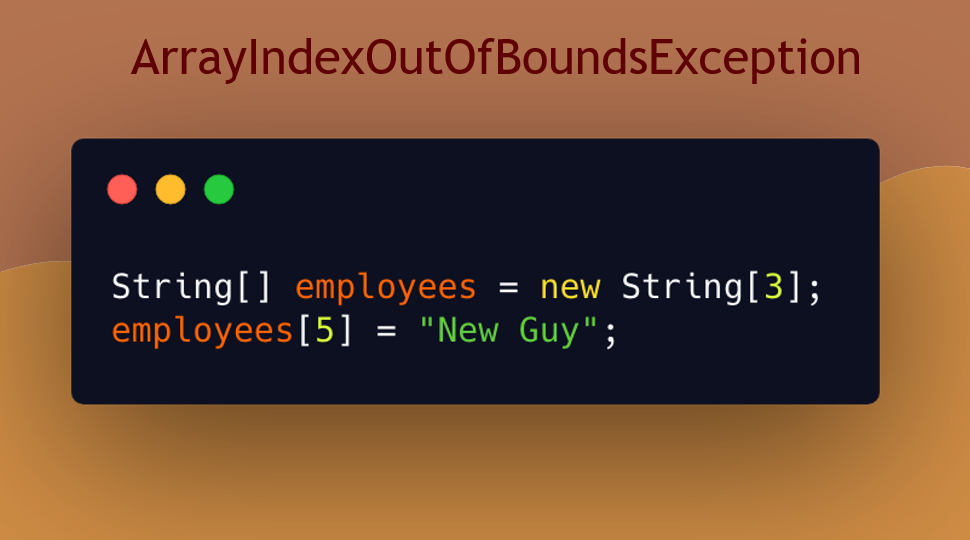In this snippet, we create an array with a length of 3, but at some point, we're trying to access index of 5. This will generate an `ArrayIndexOutOfBoundsException`.

So, if you ever see this, it means you're trying to access an index that is beyond the length of the array.

We also see this same error manifest in loops when you're off by 1.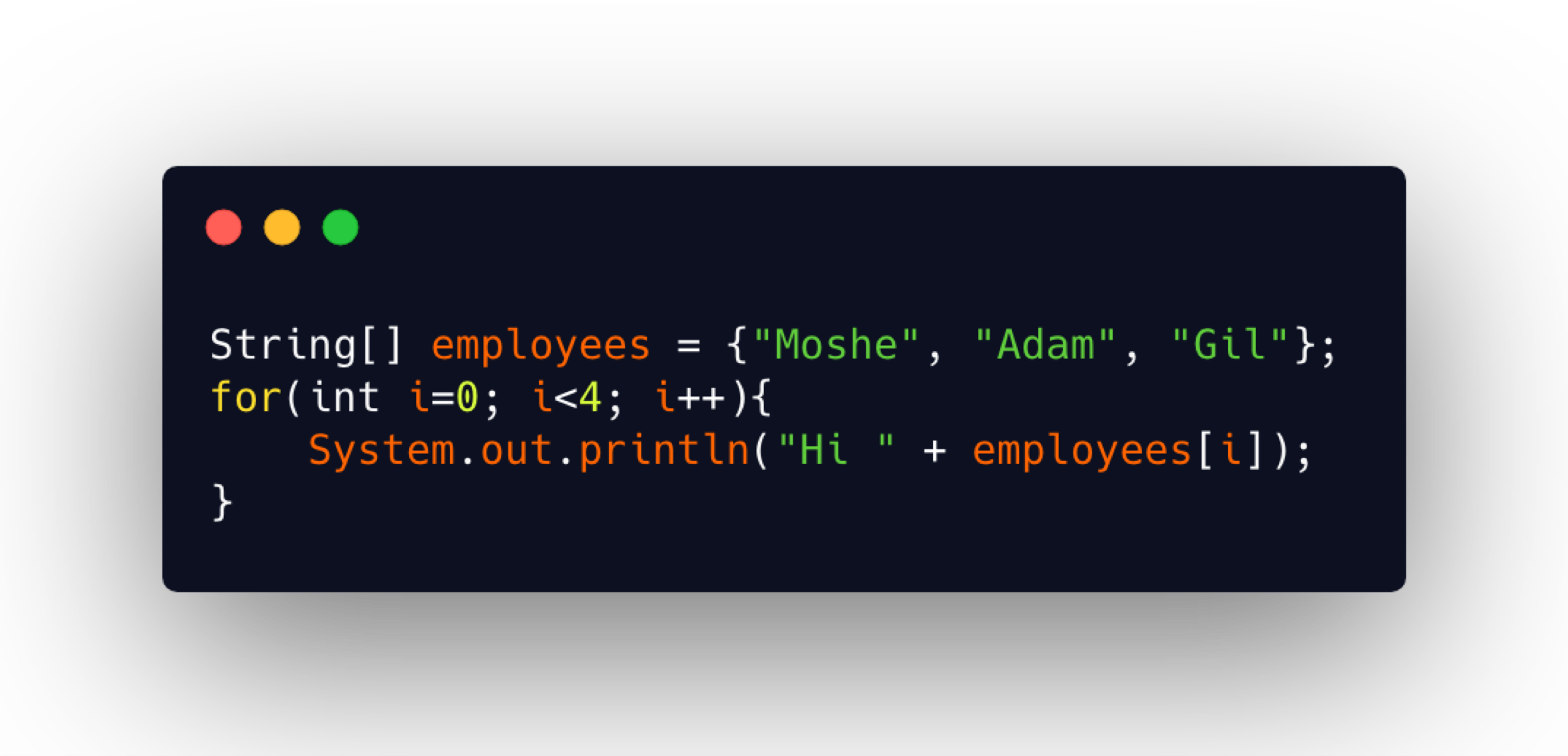This is where the loop iterates 1 too many or 1 too few times. And this happens because sometimes we get a little confused with the index starting at 0 and then the condition statement. If you’re over by 1, this will also throw an `ArrayIndexOutOfBoundsException`.

### # Arrays of Objects

So far, the only type of array we've worked with has been an `int` array, but arrays are not limited to the `int` data type. They can hold any kind of data types, even objects.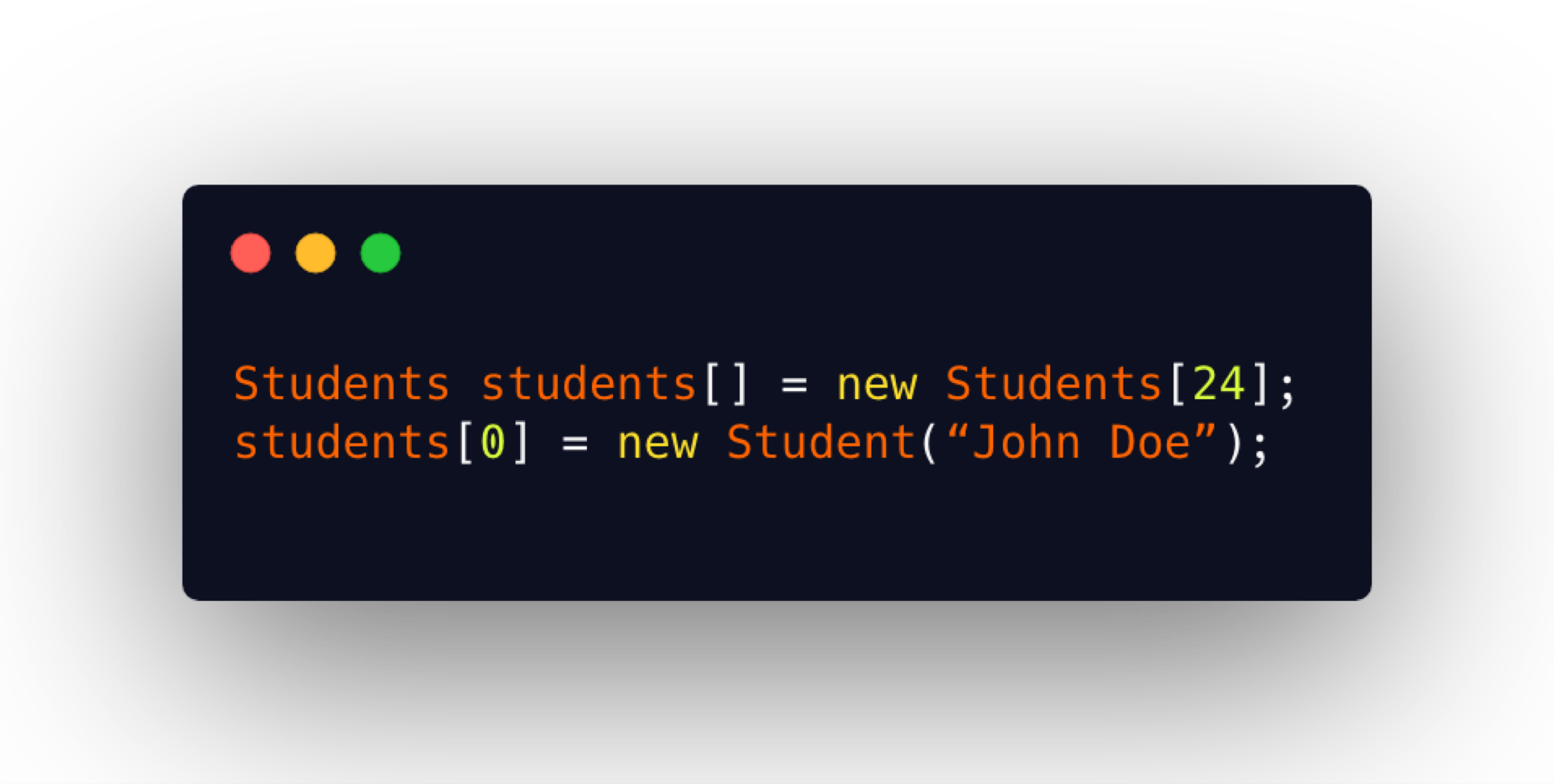In this example, we have created an array of students and we assign a new instantiation of student to the first element.

### # Multidimensional Arrays

You can also declare an array of arrays. This is called a multidimensional array and it's where the elements of an array are arrays themselves.

A two-dimensional array is like a table which holds multiple sets of data in rows, in columns.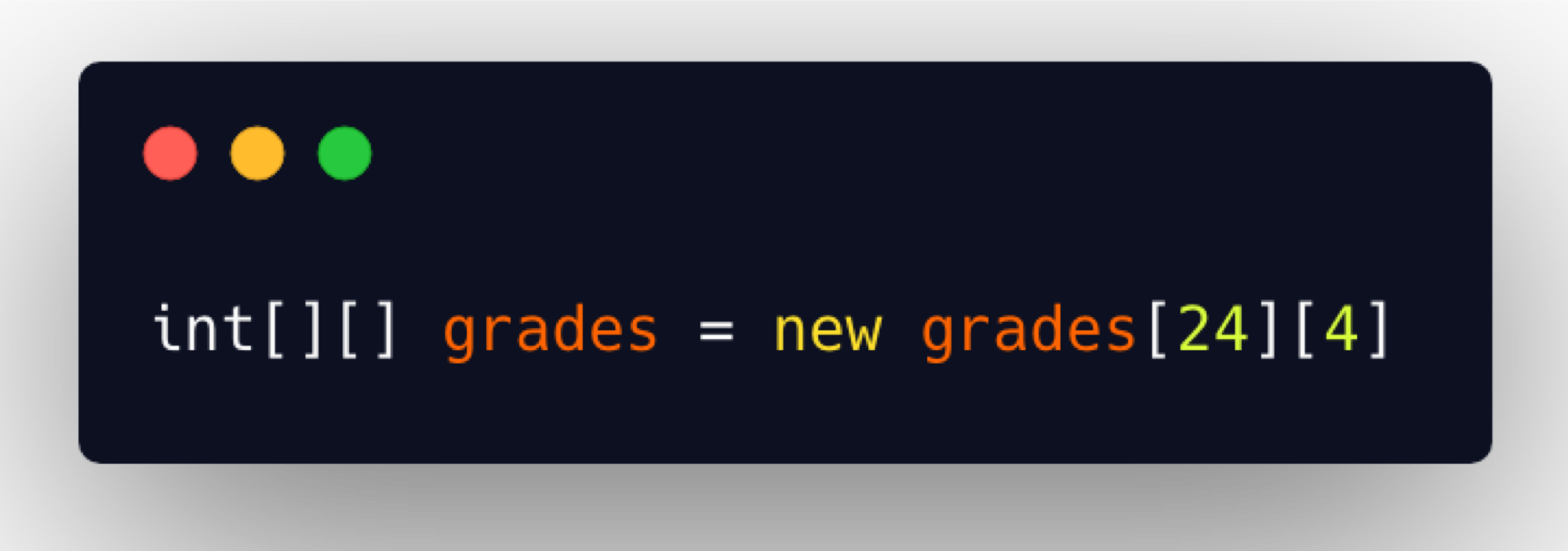This declaration is of an array that contains 2 lengths.

• The 1st one indicates the number of rows.
• The 2nd one indicates the number of columns.

In an example like this, we have a row for a student and the columns for their 4 grades. So, there will be 24 students, each having 4 grades each.

I won't go into any more detail on multidimensional arrays because it's so rarely used. I just wanted you to know that it exists should you ever need it.

## Optional Independent Exercise

For your assignment for this chapter, you're going to do a day of the week array.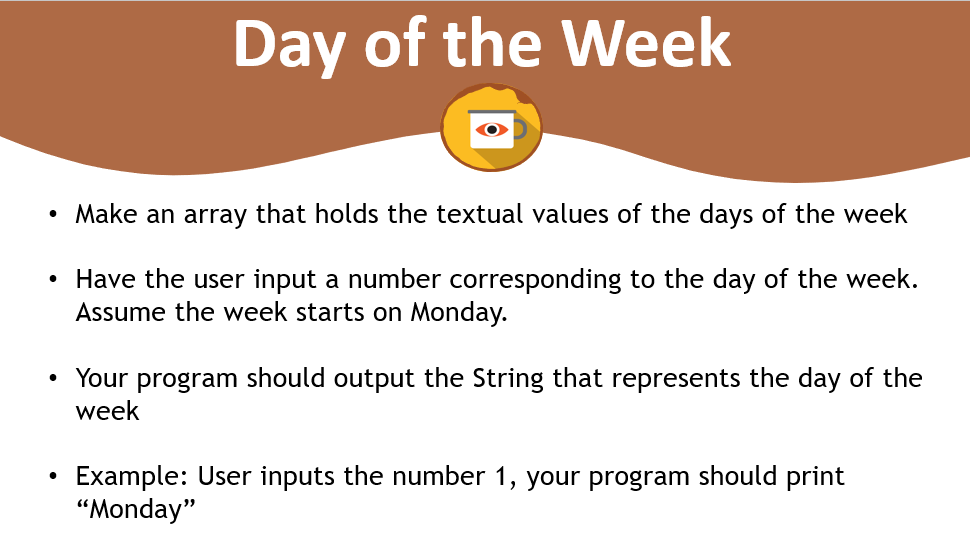Make an array that holds its actual values of the days of the week, and then have the user input a number corresponding to some day of the week. And assume that the week starts on Monday.

Your program should output the String that represents the day of the week that corresponds to the number that the user input. For example, if the user inputs the number 1, your program should print “Monday”.

This is an optional exercise. I hope that you'll give it a try.

Good luck.

Solution

Programming can be done many different ways, but here’s my solution.